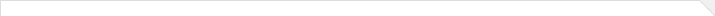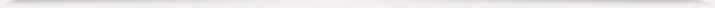报告题目： Singular kinetic equations and applications 报 告 人： 张希承 教授 报告人所在单位： 武汉大学 报告日期： 2021-10-15 报告时间： 13:30--14:30 报告地点： 腾讯会议账号：457 650 717 密码：200433 报告摘要： `In this paper we study singular kinetic equations in \$\mR^{2d}\$ by paracontrolled distribution method introduced in \cite{GIP15}. We first develop paracontrolled calculus in the kinetic setting, and then use it to establish the global well-posedness for linear singular kinetic equations under the assumptions that the products of singular terms are well-defined. We also demonstrate how the required products can be defined in the case that singular term is a Gaussian random field by probabilistic calculation. Interestingly, although the terms in the zeroth order Wiener chaos of regularization approximation are not zero, they converge in suitable weighted Besov spaces and thus no renormalization is required. As applications the global well-posedness for a nonlinear kinetic equation with singular kernels is obtained by the entropy method. Moreover, we also solve the martingale problem for nonlinear kinetic distribution dependent stochastic differential equations with singular drifts. (This is a joint work with Zimo Hao, Rongchan Zhu and Xiangchan Zhu) `10-15海报.pdf 本年度学院报告总序号： 239